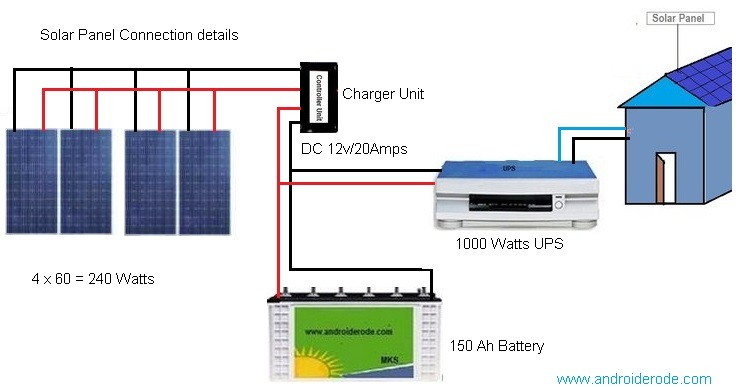Suppose if you want..
1. light load 4 nos ( old type 40 Watts type light)
2. Ceiling Fan 4 nos.
3. LED TV 42 Inch = 1.
4. Set-top Box = 1.
5. Desktop Computer with 19″ monitor.
1. 2 x 40 Watts = 200 Watts.
2. 5 x 60 Watts = 300 Watts.
3. 1 x 80 Watts = 80 Watts.
4. 1 x 5 Watts = 5 Watts.
5. 1 x 200 Watts 200 Watts.YOUR TOTAL ELECTRICAL WATTS = 785 Watts.
So select your home UPS like sine wave inverter type ( Noice and fast switching for your PC)
SELECT YOUR BACKUP TIME IN HOUR.
If you want backup time for one hour and above mentioned all items at a same time.
Battery voltage = 12 V
Battery Current = 100 Ah.
Total power by the battery is…
12 x 100 = 1200 Watt hours.Is your consumption is 400 Watts…
This battery will come upto 3 Hours like.
If your requirement is 2 hour add another one 12/100 Ah battery with parallel.
HOW TO SELECT SOLAR PANEL FOR ABOVE MENTIONED 1000 WATTS HOME UPS.
First count how many battery are you connected with your home ups.
Suppose my calculation is one battery and capacity is 12V / 100Ah.
12V / 100 Ah battery charging current should be one / ten times of its total Ah.
Calculation:
12/100 Ah
100 x (1/10) = 10 Amps.
Power is
P=VxI,
P= 12 X 10
P= 120 Watts.
So we will connect 2 numbers of 60 Watts solar panels in parallel. Because each panel capacity is 12 V / 5 Amps.
In addition we will connect another one of battery like 12V/100Ah
Total battery is 2 nos.
Total 200 Ah charging current is..
200 x (1/10) = 20 Amps.
Power is
P=VxI,
P= 12 X 20
P= 240 Watts.
At this condition you can add 4 numbers of solar panel like 12/5 Amps model in parallel.